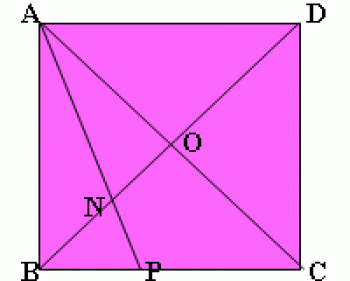Games
Problems
Go Pro!

Problems > High School Math > 2003

## An Increasing Function for the New Year

Let f be a function from Z+ to Z+ where Z+ is the set of positive integers, such that f satisfies these two conditions:

(1) f(n+1) > f(n); that is, f is strictly increasing

And

(2) f(n+f(m)) = f(n)+m+1

Find all values of f(2003)

## Evaluate This Indefinite Integral

What is the integral,

òdx/(x + sqrt(1-x²))

?

## A Geometrical DiversionThe diagonals of a square meet at O.

The bisector of angle OAB meets

BO and BC at N and P respectively.

The length of NO is 24.

How long is PC?

## Interesting Integer Sequences

Let A be the set of all possible finite sequences (n0, n1, ..., nk) of integers such that,

for each i = 0, 1, ..., k

i appears in the sequence ni times.

Here are some sequences in set A:

1,2,1,0

2,0,2,0

2,1,2,0,0

3,2,1,1,0,0,0

4,2,1,0,1,0,0,0

k-3,2,1,0,0,...,1,0,0,0

Are there other sequences in set A? If so, what are they?

Now prove it.

## Another Increasing Function

Maybe you remember that back in January 2003, I offered you an increasing function that met two criteria (click the problem archives to see it). This month, I will challenge you with another increasing function that meets two criteria...

Let f map positive integers to positive integers with the conditions:

i) f(n+1) > f(n)

ii) f(f(n)) = 3n

Find f(955).

## Oh, You Can't Be Serious!

If 4x + 4-x = 7, then what is 8x + 8-x?

## Strange Sum

Let A1776 be the set { 1, 1/2, 1/3, ..., 1/1776 }

Remove any two elements, say a and b, from A1776, and replace them with the single number ab+a+b to form set A1775.
Continue in this manner, until you have performed 1775 such operations, to form set A1, which contains a single element.

What is this element?

Prove it!

## A Little Number Theory to Begin the School Year

Prove that if p and p²+8 are prime then so is p³+4.

## It's Cotton Candy for the Mind

Simplify the infinite product (1+x)(1+x2)(1+x4)(1+x8)(1+x16)...,
given |x| < 1.

## Party Hardy

(Part A): There are 100 people in a ballroom. Every person knows at least 67 other people (and if I know you, then you know me). Prove that there is a set of four people in the room such that every two from the four know each other. (We will call such a set a "clique.")

(Part B): Two people in the room are Joe and Grace, who know each other. Is there a clique of four people which includes Joe and Grace?

(Part C): Oops, I miscounted. There are actually 101 people in the room, But it's still true that each knows at least 67 others. That can't make a difference, can it?

## A Strong Will

A father in his will left all his money to his children in the following manner:

\$1000 to the first born and 1/10 of what then remains, then

\$2000 to the second born and 1/10 of what then remains, then

\$3000 to the third born and 1/10 of what then remains, and so on.

When this was done each child had the same amount. How many children were there?Like us on Facebook to get updates about new resources